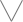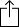C
D
E
F
G
A
B
Chord
Scale
3nps
CAGED
None
Notes
Degrees
Intervals
None

1
2
3
4
5
6
7
8
9
10
11
12
13
14
15
16
17
18
19
20
21
22
23
24
E
B
G
D
A
E
F
G
G
A
A
B
B
C
D
D
E
E
F
G
G
A
A
B
B
C
D
D
E
E
C
D
D
E
E
F
G
G
A
A
B
B
C
D
D
E
E
F
G
G
A
A
B
B
A
A
B
B
C
D
D
E
E
F
G
G
A
A
B
B
C
D
D
E
E
F
G
G
E
E
F
G
G
A
A
B
B
C
D
D
E
E
F
G
G
A
A
B
B
C
D
D
B
B
C
D
D
E
E
F
G
G
A
A
B
B
C
D
D
E
E
F
G
G
A
A
F
G
G
A
A
B
B
C
D
D
E
E
F
G
G
A
A
B
B
C
D
D
E
E

# C Man Gong(慢宮調) for Guitar

C Man Gong(慢宮調) is a pentatonic scale. Pentatonic scales have 5 notes, "penta" comes from the greek word "Pentateuch" which means 5.

The C Man Gong(慢宮調) scale is derived from the C Phrygian mode by taking scale degree 1, 3, 4, 6 and 7 of the scale.

C
E
F
A
B
Cm3
W
m3
W
W
Play scaleand then